# 4 Interesting Data Structures and Algorithms

| 7 min. (1347 words)

Raygun is built by a curious team of developers just like you – they like to share their thoughts and ideas from time to time. Alex shares his thoughts on data structures.

Quadtrees are an intuitive spacial partitioning structure. They can be used to make large sets of entities easily searchable by subdividing the data space recursively into quadrants.

Quadtrees can be understood easily when you see how they are constructed.

They are constructed by taking a candidate area which bounds all the entities and defining a rectangle around it. The rectangle is this recursively divided into smaller and smaller quadrants until each quadrant has less than a given amount of entities. Thus a tree like structure with each non-leaf node having 4 children is made.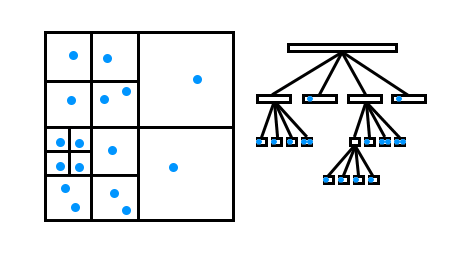Here you can see data points (in blue) within a quadtree. On the left is a spacial representation on the right is the tree structure.

The real magic of the Quadtree is being able to find entities quickly based on their location and even do things like find which two objects are closest to each other. It can do this by using the implicit information given by the spacial nature of the tree.

If a search is being made on an area outside of a high level quadrant we know that all the data-points within the child quadrants are not in the search space and can be ignored. Thus when finding data-points in the tree the vast majority of entities do not need to be considered.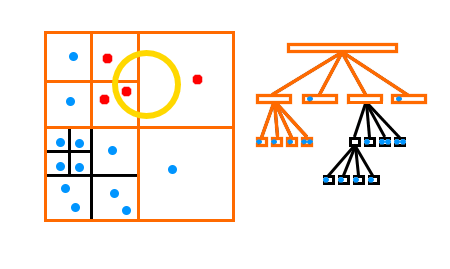Here you can see that because the search query (in yellow) doesn’t intersect with any of the child quadrants in the bottom left, the bottom left entities don’t need to be checked, only the datapoints in red and the quadrant boundaries in orange need to be checked.

## Hyperloglog

The Hyperloglog algorithm, is a way of maintaining a count of the number of discrete elements in a set without having to consider every element already in the set when considering a new element.

It does this  to get an estimate on the number of distinct objects which exist.

The basic gist of the algorithm is that by using the statistical likelihood that certain ranges of numbers are present we can estimate the number of distinct objects which exist.

If for each object in the set we can generate a fixed length hash we can use the value and our knowledge about likelihood of that value occurring given that it was generated from a normally distributing hash function to help us determine the total number of objects we have.

For example say we are given an 8 bit hash of an object, the likelihood we generate a value of or less than 00000111 is quite small. Another way to look at is is, the likelihood of generating a hash value with a large number of consecutive zeros is quite small.

I our 8 bit example a number with 5 consecutive zeros has a 1/32 chance of being generated. Thus we could assume that if we see a hash value with 5 consecutive zeros in a stream of hash values there is a good chance there is at least 32 numbers in our stream.

Of course using a single number to determine the total number of objects that we have is going to introduce a high amount of variance (what if the first hash we generate is 0000000? that would imply that we have seen more than 256 distinct objects), that’s why Hyperloglog stores many lowest hash values in different buckets and averages are taken across them in order to determine a more accurate estimate.

This has just covered one of the underlying principles of the algorithm, if you want to find out more you can read about it in more detail here: http://research.neustar.biz/2012/10/25/sketch-of-the-day-hyperloglog-cornerstone-of-a-big-data-infrastructure/

## Genetic Algorithms

Genetic Algorithms are a solution to optimisation problems inspired by biological evolutionary adaption.

Genetic algorithms usually involve a generational approach to evolving a set of genetic information to achieve the best result at a specific task.

The task is usually posited as a fitness function, which returns a value determining how well the actor achieved.

For example one may wish to evolve a solution for optimising the distribution of resources. The fitness function may return the cost of the distribution when given the genetic information of the actor.

Genetic algorithms can range from being very complicated to very simple. Some may have genomes with a fixed number of genes.

For instance (in an absurdly simple example) an algorithm evolving the optimum angle to throw a ball may have only the angle of the throw as it’s genetic code.

More complicated algorithms could have instructions encoded within the genetic code, such as a simple syntax. In this case behaviours could be evolved.

The fitness function is the other core compontent of Genetic Algorithms, it takes the genetic information and runs a calculation or simulation to determine how fit the genetic code is. It can be as simple as a small maths equation or as complex as a physical simulation.

Once fitness has been determined for each participant the weaker participants are removed from the gene pool and the strong ones are cross bred (have their genetic code swapped with other succeeding actors) and randomly mutated. This allows for additional convergence on the true optimal solution without getting stuck in a local minima.

## A*

A* is a graph traversal algorithm designed to efficiently find the shortest path between any two points on a connected graph. It’s used for many applications including route planning for navigation systems.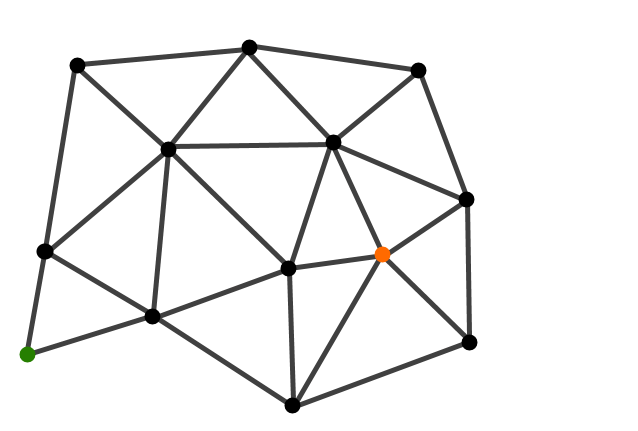Here is a graph we want to traverse. Starting at the orange dot we want to find the shortest route to the green dot.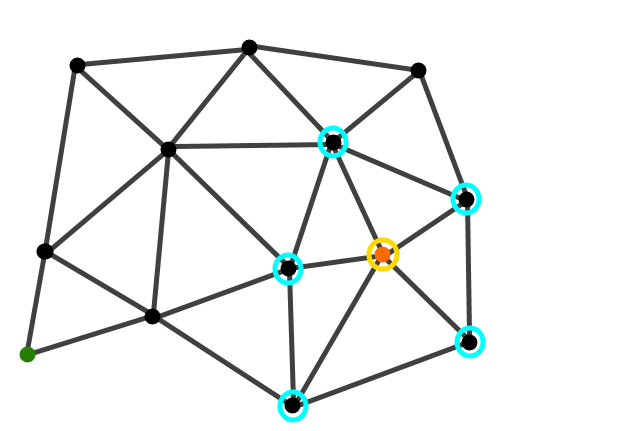Form the starting node we find all connected neighbours.

A* works by coming up with a good guess at what the best way to traverse a graph is and exploring in that direction first. It does this by guessing how far any node is from the goal node. This could be a naive distance calculation between the goal and the evaluated node. Given this estimate the algorithm is likely to expand more directly towards the goal which is desirable except for in highly misleading graphs where there may be dead ends when routes taken towards the goal are taken.

This is what makes it distinct from a naive Dijkstra algorithm which explores in every direction until the target is found.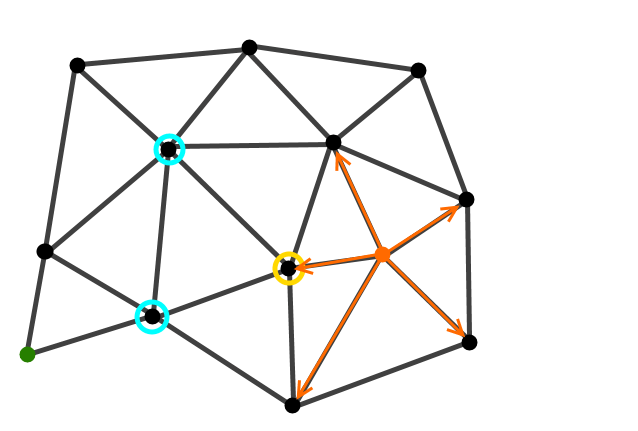We assign a parent node to the expanded nodes. We then nominate a node out of our new edge nodes (circled in yellow).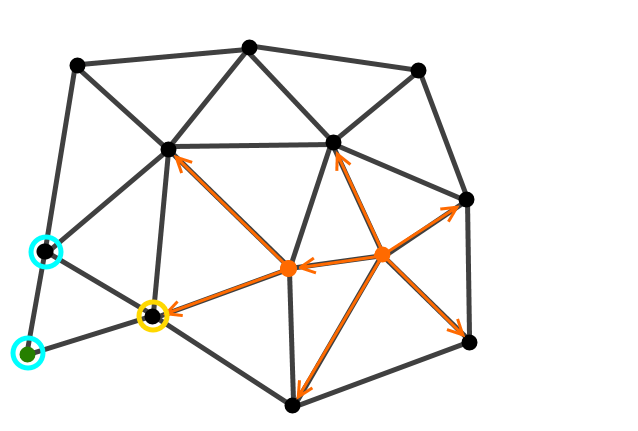As the process repeats we can see large areas of the graph go unexplored because the heuristic chooses good candidate nodes to expand upon.

Given a starting position on a graph and a target position the algorithm evaluates the nodes nearest the start node for two things. Firstly how far the node is from the start node and secondly how far the node is from the goal, the problem is that unless we know the final path we don’t actually know how far away the goal node is. This is where the heuristic comes in. Instead of calculating the distance to every node in the graph like Dijkstra’s algorithm, it simply takes a guess, this could be based on the co-ordinate distance between the evaluated node and the goal node or some other type of guess, all it has to do is not be longer than the actual distance to the goal node. After evaluating the nodes they are all placed in a heap where the one with the lowest heuristic + pathlength will be removed from. After this node is popped

You can read more about the details of A* and play with some of the interactive diagrams here: http://www.redblobgames.com/pathfinding/a-star/introduction.html

Did you know Raygun saves you time and helps you build better software?

Try Raygun for free today and discover why so many developers across the world use Raygun with their apps.